# COMSOL® 中声固耦合的建模

2019年 6月 12日

### 可用于声固耦合分析的物理场接口• 压力声学
• 压力波在黏性边界层之外的流体域中并且处于静止的条件下
• 热黏性声学
• 在声学的详细建模过程中，要包括壁附近的边界层，其中黏性损失和热传导不可忽略
• 气动声学
• 在静止环境下的声学改变就意味着流动，也称为对流声学
• 超声
• 使用不连续的Galerkin方法可以在比波长长度更大的距离里传播声信号
• 几何声学
• 高频声学，其声学波长远小于其几何特征### 使用预定义的多物理场接口对声固耦合进行建模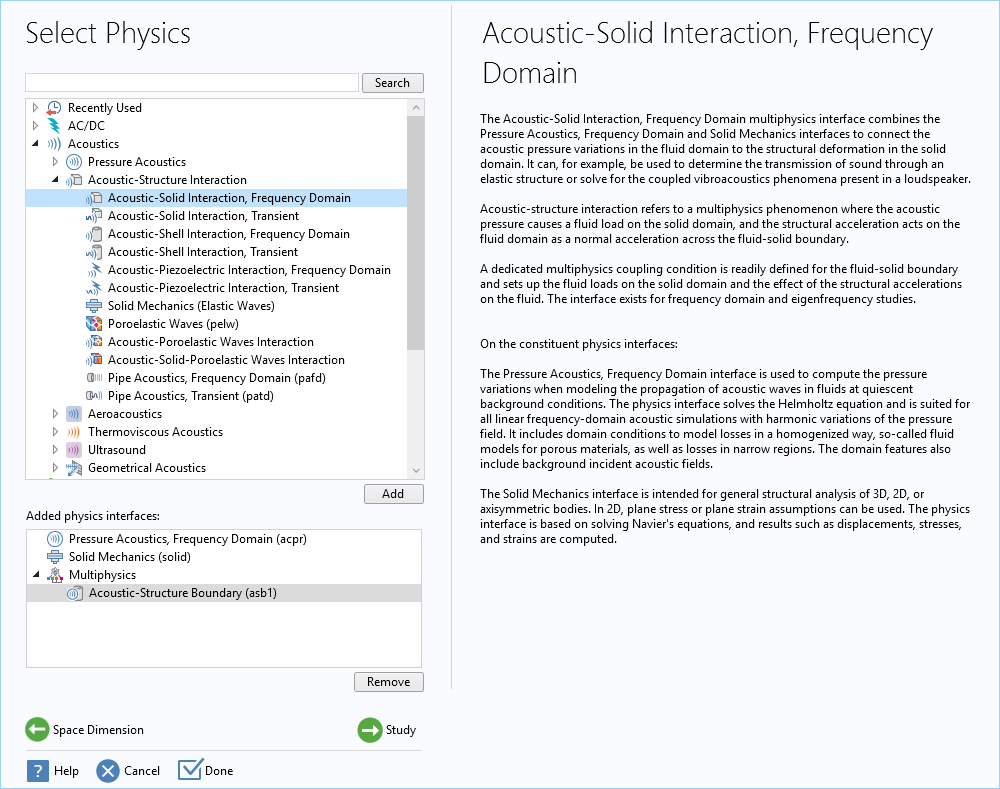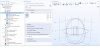### ASI的耦合方法

-\textbf
{n} \cdot \Big(-\frac{1}{\rho_c}(\nabla p_t – q_d)\Big) = -\textbf{n}
\cdot \textbf
{u}_{tt}
\textbf{F}_A = p_t\textbf{n}

-\textbf{n} \cdot \Big(-\frac{1}{\rho_c}(\nabla p_t – q_d)\Big)_{up} = -\textbf{n} \cdot \textbf{u}
_
{tt}
-\textbf{n} \cdot \Big(-\frac{1}{\rho_c}(\nabla p_t – q_d)\Big)_{down} = -\textbf{n} \cdot \textbf{u}_{tt}
\textbf
{F}
_A = (p_t,down – p_t,up)\textbf
{n}

• 声学结构边界
• 热黏性声学结构边界
• 气动声学结构边界
• 声-孔边界
• 多孔结构边界

### 通过手动添加物理接口和耦合来对ASI进行建模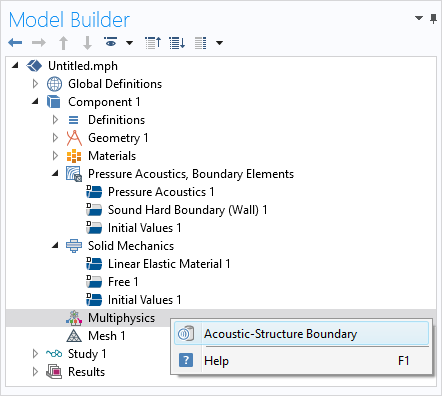### 示例：对集总接收器进行建模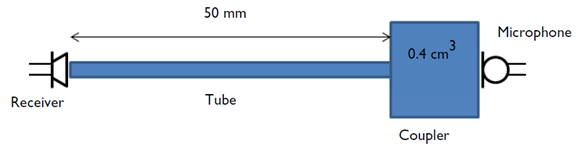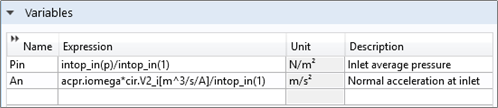intop_in()在细管的入口表面上定义了一个积分算子，其中，p 是声压，acpr.iomega 是复合角频率，和 cir.V2_i是 SPICE 接收器输出处的电流。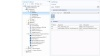### 解决大型ASI模型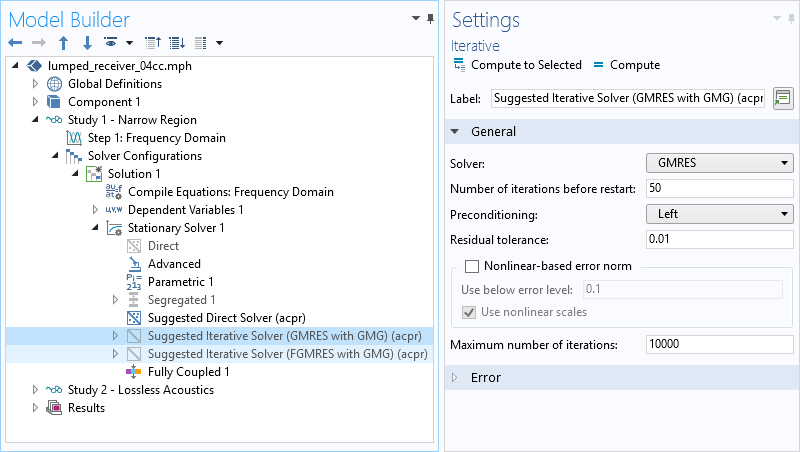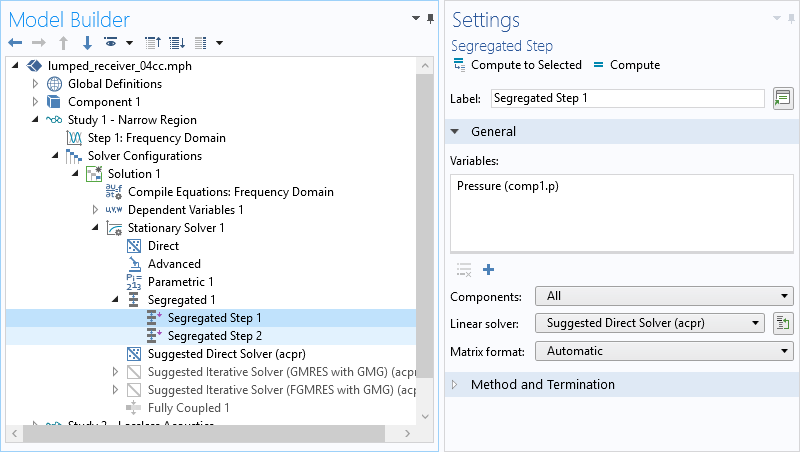### 下一步

• 在 COMSOL 博客上了解有关声学建模的更多信息：

#### 评论 (2)

##### 留言##### Jie Li
2022-03-302022-08-29